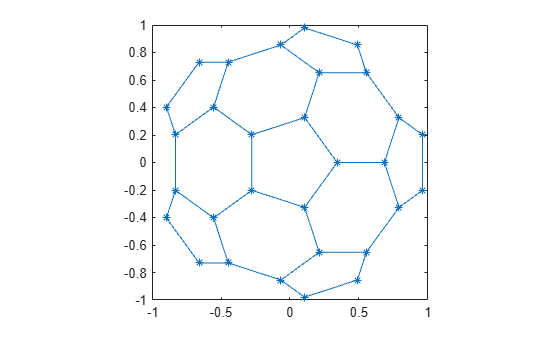# gplot

Plot nodes and edges in adjacency matrix

## Syntax

``gplot(A,XYCoords)``
``gplot(A,XYCoords,LineSpec)``
``[x,y] = gplot(A,XYCoords)``

## Description

example

````gplot(A,XYCoords)` plots a graph of the nodes and edges defined in the adjacency matrix `A` at the coordinates specified in `XYCoords`. The adjacency matrix `A` is an `n`-by-`n` matrix, where `n` is the number of nodes. `XYCoords` is an `n`-by-`2` matrix specifying xy-coordinates for each node.```

example

````gplot(A,XYCoords,LineSpec)` additionally uses `LineSpec` to specify the line type, marker symbol, and color to use in the plot. For example, `gplot(A,XY,'r-*')` uses red lines for edges and red asterisks for nodes.```
````[x,y] = gplot(A,XYCoords)` returns the `NaN`-delimited vectors `x` and `y` without generating a plot. Use `x` and `y` to generate a plot at a later time using `plot(x,y)`. ```

## Examples

collapse all

Plot half of the carbon-60 molecule, placing asterisks at each node.

```k = 1:30; [B,XY] = bucky; gplot(B(k,k),XY(k,[1 2]),'-*') axis square```## Input Arguments

collapse all

Adjacency matrix, specified as a matrix. `A` describes the connections between the nodes in the graph by the location of nonzero values. If node `i` and node `j` are connected, then `A(i,j)` or `A(j,i)` is nonzero; otherwise, `A(i,j)` and `A(j,i)` are zero.

Example: `A = ones(5)` is the adjacency matrix of a graph with five nodes where each node is connected to all the others.

Example: `A = [0 1 1 1; 1 0 0 0; 1 0 0 0; 1 0 0 0]` is the adjacency matrix of a graph with four nodes where one node connects to the other three.

Data Types: `single` | `double`

xy-coordinates of nodes, specified as an `N`-by-`2` matrix. Each row in `XYCoords` defines the coordinates for one node in the graph, so `XYCoords(i,:) = [x(i) y(i)]` gives the coordinates for node `i`.

Example: `XYCoords = [1 2; 3 4]` plots one node at (1,2) and a second node at (3,4).

Line style, marker, and color, specified as a character vector or string containing symbols. The symbols can appear in any order. You do not need to specify all three characteristics (line style, marker, and color). For example, if you omit the line style and specify the marker, then the plot shows only the marker and no line.

Example: `'--or'` is a red dashed line with circle markers

Line StyleDescriptionResulting Line
`'-'`Solid line`'--'`Dashed line`':'`Dotted line`'-.'`Dash-dotted lineMarkerDescriptionResulting Marker
`'o'`Circle`'+'`Plus sign`'*'`Asterisk`'.'`Point`'x'`Cross`'_'`Horizontal line`'|'`Vertical line`'s'`Square`'d'`Diamond`'^'`Upward-pointing triangle`'v'`Downward-pointing triangle`'>'`Right-pointing triangle`'<'`Left-pointing triangle`'p'`Pentagram`'h'`HexagramColor NameShort NameRGB TripletAppearance
`'red'``'r'``[1 0 0]``'green'``'g'``[0 1 0]``'blue'``'b'``[0 0 1]``'cyan'` `'c'``[0 1 1]``'magenta'``'m'``[1 0 1]``'yellow'``'y'``[1 1 0]``'black'``'k'``[0 0 0]``'white'``'w'``[1 1 1]`## Output Arguments

collapse all

Node coordinates, returned as vectors. `x` and `y` contain the same information as `XYCoords`, but in a different format that is suitable for plotting with the command `plot(x,y)`. The line segments defined in `x` and `y` are separated with `NaN` values.